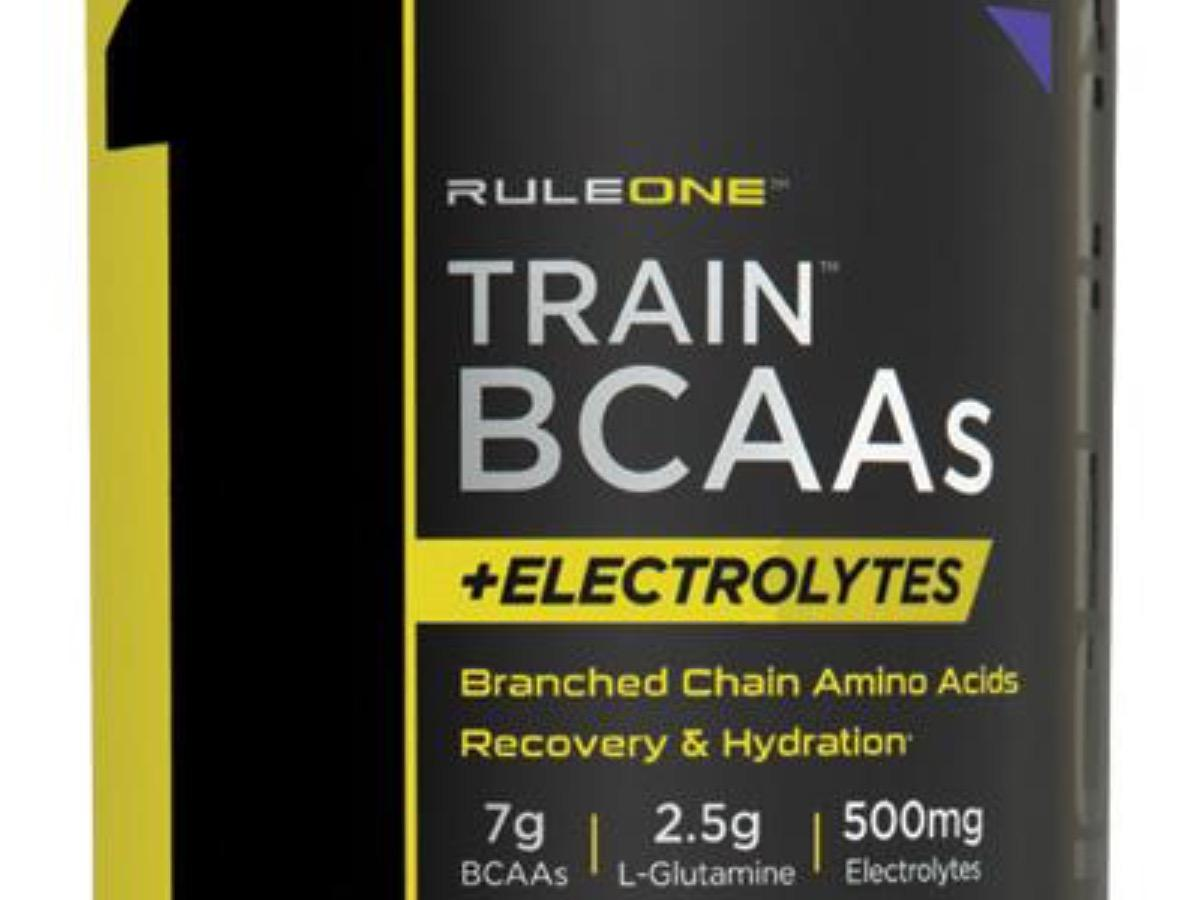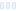# R1 Train, Amino Acid Formula, Blue Raspberry

## Rule 1

Main info:

R1 Train, Amino Acid Formula, Blue Raspberry
Rule 1
1 scoop
5 Calories
1 g
0 g
0 g

0 g
0 mg
0 g
160 mg
0 g
0 g

Percent calories from...
Nutrition Facts
For a Serving Size of (g)
How many calories are in R1 Train, Amino Acid Formula, Blue Raspberry? Amount of calories in R1 Train, Amino Acid Formula, Blue Raspberry: Calories Calories from Fat (%)
% Daily Value *
How much fat is in R1 Train, Amino Acid Formula, Blue Raspberry? Amount of fat in R1 Train, Amino Acid Formula, Blue Raspberry: Total Fat
How much sodium is in R1 Train, Amino Acid Formula, Blue Raspberry? Amount of sodium in R1 Train, Amino Acid Formula, Blue Raspberry: Sodium
How much potassium is in R1 Train, Amino Acid Formula, Blue Raspberry? Amount of potassium in R1 Train, Amino Acid Formula, Blue Raspberry: Potassium
How many carbs are in R1 Train, Amino Acid Formula, Blue Raspberry? Amount of carbs in R1 Train, Amino Acid Formula, Blue Raspberry: Carbohydrates
How many net carbs are in R1 Train, Amino Acid Formula, Blue Raspberry? Amount of net carbs in R1 Train, Amino Acid Formula, Blue Raspberry: Net carbs
How much protein is in R1 Train, Amino Acid Formula, Blue Raspberry? Amount of protein in R1 Train, Amino Acid Formula, Blue Raspberry: Protein
Vitamins and minerals
How much Vitamin C is in R1 Train, Amino Acid Formula, Blue Raspberry? Amount of Vitamin C in R1 Train, Amino Acid Formula, Blue Raspberry: Vitamin C
Fatty acids
Amino acids
* The Percent Daily Values are based on a 2,000 calorie diet, so your values may change depending on your calorie needs.Loading similar foods...
Note: Any items purchased after clicking our Amazon buttons will give us a little referral bonus. If you do click them, thank you!Be cool

- Zen orangeI never skip arm day

- Buff broccoli